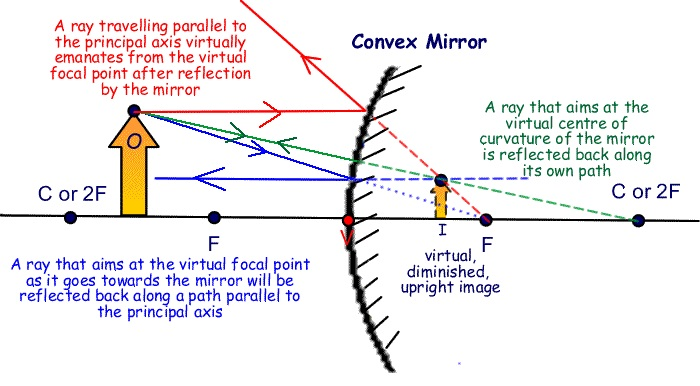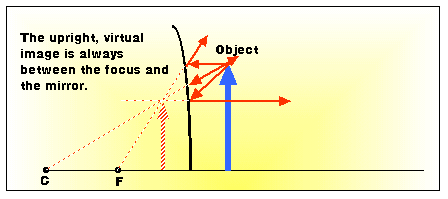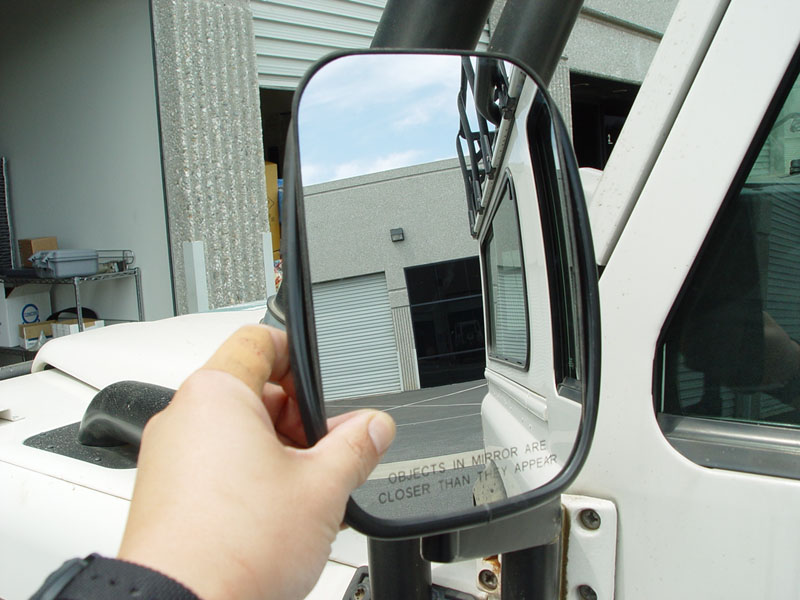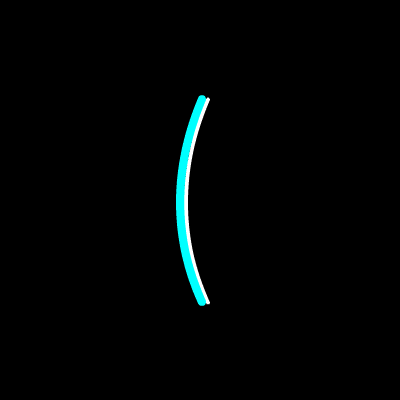CONCAVE MIRRORS!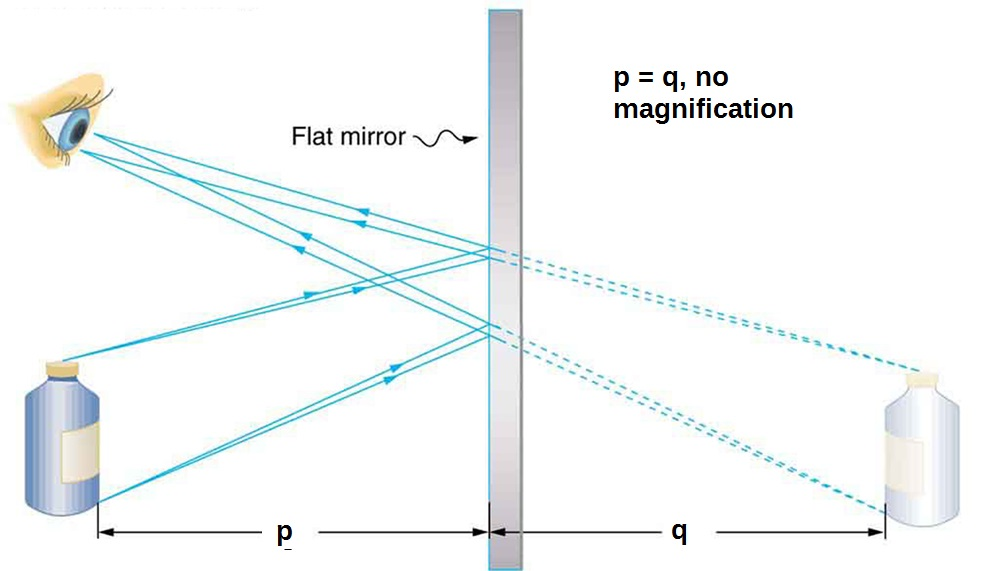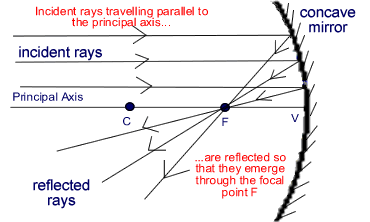Concave mirrors can form a real image, bringing light to a focus. The geometrical relation is (1/p) + (1/q) = (1/f) = (2/R), where p is the object distance, and q is the image distance, with R the radius of curvature of the mirror surface and f the focal length of the mirror.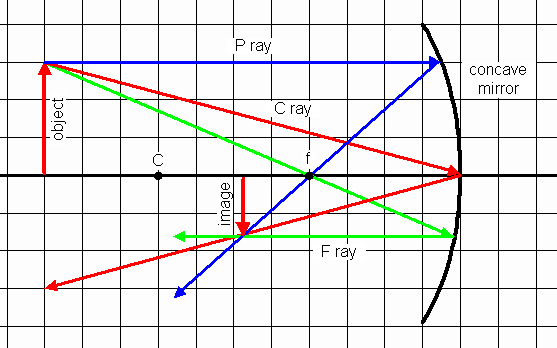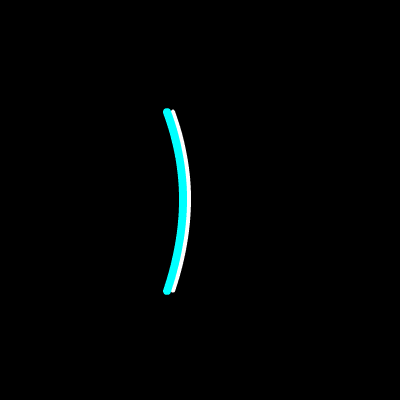To locate the image, draw any two of three key rays. (1) A ray (P) parallel to the axis of symmetry, which will be reflected through the focal point. (2) A ray (F) passing through the focal point, which will be reflected parallel to the symmetry axis, and (3) a ray (C) hitting the mirror in its center, which will be reflected just as if it had hit a flat mirror.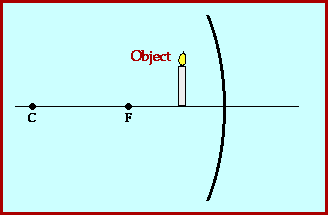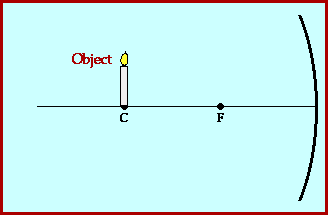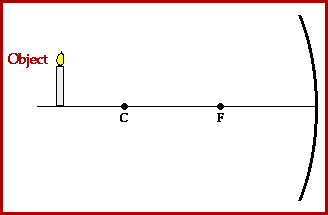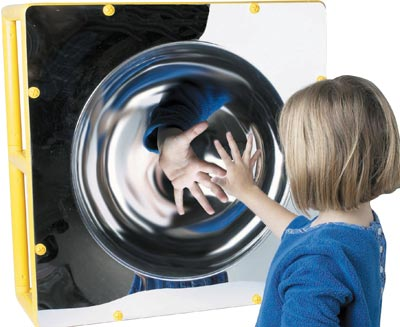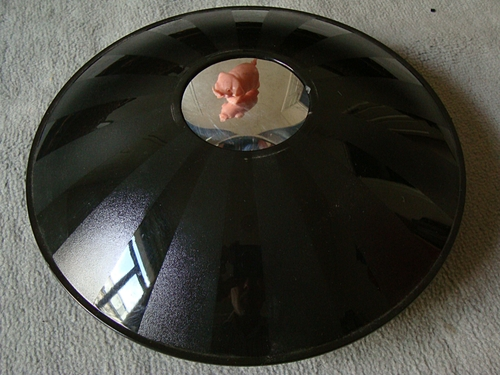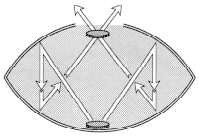For convex mirrors it's easy to keep sign conventions straight and check your answers if you just trace the rays first. Note that convex mirrors always produce a virtual image, just as plane mirrors do.